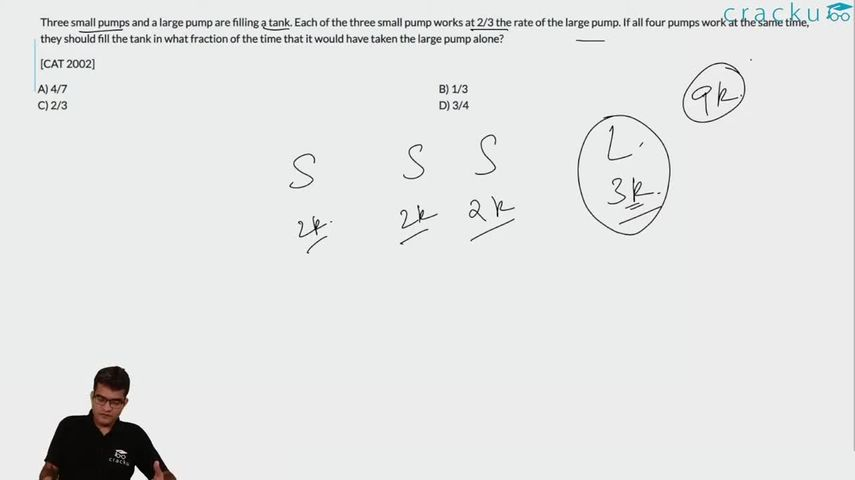Question 50

# Three small pumps and a large pump are filling a tank. Each of the three small pump works at 2/3 the rate of the large pump. If all four pumps work at the same time, they should fill the tank in what fraction of the time that it would have taken the large pump alone?[CAT 2002]

Solution

Let the work done by the big pump in one hour be 3 units.
Therefore, work done by each of the small pumps in one hour = 2 units.
Let the total work to be done in filling the tank be 9 units.
Therefore, time taken by the big pump if it operates alone = 9/3 = 3 hours.
If all the pumps operate together, the work done in one hour = 3 + 2*3 = 9 units.
Together, all of them can fill the tank in 1 hour.
Required ratio = 1/3

### View Video Solution